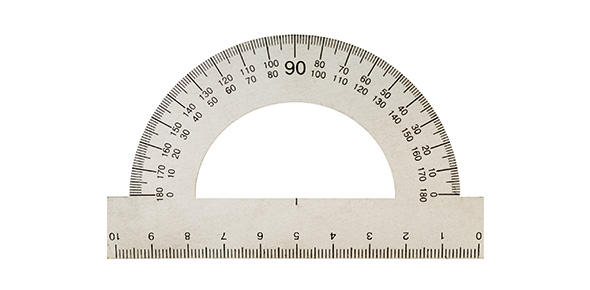# (1.) Physics Hl/Sl. Physical Measurement And Kinetics

30 Questions | Attempts: 61
ShareSettingsQuiz on physics sections 1 and 2, focusing on definitions. Don't screw up the definition questions! You get free points if you just learn them.

• 1.
A derived unit is a unit derived from the combination of [...] units
• 2.
An accurate experiment is one that has a small [...] error
• A.

Percentage

• B.

Systematic

• C.

Random

• 3.
A graph is extended in order for an intercept to be found. This process is known as ...
• A.

Integration

• B.

Interpolation

• C.

Extrapolation

• 4.
The process of assuming that the trend line applies between two points is known as [...]
• 5.
Displacement (as a kinematic concept) can be defined as "the distance moved in a particular direction"
• A.

True

• B.

False

• 6.
Velocity is the rate of change of [...]
• 7.
Speed is the rate of change of [...]
• 8.
Acceleration is the rate of change of [...]
• 9.
Out of the concepts just defined (displacement, velocity, speed, and acceleration), two are scalars and two are vectors.
• A.

True

• B.

False

• 10.
The [...] velocity of an object is its velocity at a specific instant of time
• A.

Average

• B.

Constant

• C.

Terminal

• D.

Instantaneous

• 11.
An object is in a uniform gravitational field, accelerating vertically. Air resistance may be neglected. The object is thus in [...]
• 12.
A free-falling object has a net force on it that is equal to zero. The downward force of gravity has come to equal the upward force of drag. What has the object achieved?
• A.

Constant acceleration

• B.

Terminal velocity

• C.

Strobe photography

• 13.
The origin of all everyday forces is either gravitational or [...]
• 14.
Up to an elastic limit, the extension, x, of a spring is proportional to the tension force, F. Who's the guy behind this law?
• A.

Newton

• B.

Rutherford

• C.

Peter Stråe

• D.

Hooke

• 15.
If the resultant force on an object is zero, then it is said to be in [...]
• 16.
Correct statement of Newton's second law: The resultant force is proportional to the rate of change of [...]
• 17.
"The force that opposes the relative motion of two surfaces and acts along the surfaces" Which force is being defined?
• A.

Tension

• B.

Friction

• C.

Compression

• D.

Lift

• 18.
Air resistance and drag are frictional forces
• A.

True

• B.

False

• 19.
The opposite of the tension force is the [...] force.
• 20.
Graviational force is the force between objects as a result of their ...
• A.

Inertia

• B.

Momentum

• C.

Weights

• D.

Masses

• 21.
The amount of matter contained in an object is the object's [...]
• 22.
The impulse can be defined as ...
• A.

The change in kinetic energy

• B.

The result of an inelastic collision

• C.

The change of momentum

• D.

The change of weight

• 23.
A collision in which no mechanical energy is lost is known as [...]
• 24.
Momentum is conserved in a totally inelastic collision
• A.

True

• B.

False

• 25.
Kinetic energy is conserved in an inelastic collision
• A.

True

• B.

False

## Related TopicsBack to top
×

Wait!
Here's an interesting quiz for you.